# division Fractions Problems

#### Number of problems found: 104

• Ten fractionsWrite ten fractions between 1/3 and 2/3
• Fractions 4How many 2/3s are in 6?
• ChestnutsThree divisions of nature protectors participated in the collection of chestnut trees.1. the division harvested 1250 kg, the 2nd division by a fifth more than the 1st division and the 3rd division by a sixth more than the second division. How many tons of
• Product of two fractionsProduct of two fractions is 9 3/5 . If one of the fraction is 9 3/7. Find the other fraction.
• Numbers divisionWith what number should be divided mixed number 2 3/4 to get 11/12?
• Two divided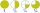Two divided by nine tenths.
• MilimetersHow many millimeters is 1/4 meters?
• Container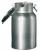How many three-quarters liters jar fill 30 liters container?
• A quotientWhat is the quotient of 3/10 divided by 2/4 as a fraction?
• Find the 11Find the quotient of 229.12 and 12.32
• Minutes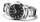Write as fraction in basic form which part of the week is 980 minutes.
• Reciprocal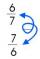Forgiven real numbers, calculate the reciprocal numbers.
• Minute hand v2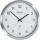In how many minutes describe the minute hand angle 60 degrees?
• Women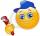In an company of 1050 employees are 2/3 women. How many women work in the company?
• The quotientThe quotient of g and 55 is the same as 279. What is g?
• What is 11What is the quotient of Three-fifths and 1 Over 10?
• Unit rateFind unit rate: 6,840 customers in 45 days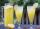How many 1/4 cup servings are in 2 and 1/3 cups of lemonade?
• Equation - inverseSolve for x: 7: x = 14: 1000
• Cake 71/3 of a cake shared with 4 people. What share of the whole cake has each people?

Do you have an interesting mathematical word problem that you can't solve it? Submit a math problem, and we can try to solve it.

We will send a solution to your e-mail address. Solved examples are also published here. Please enter the e-mail correctly and check whether you don't have a full mailbox.

Please do not submit problems from current active competitions such as Mathematical Olympiad, correspondence seminars etc...

Need help calculate sum, simplify or multiply fractions? Try our fraction calculator. Division Problems. Fraction Word Problems.